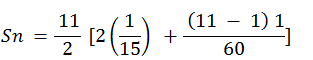Newbie

# Find the sum of the A.P. 1/15, 1/12, 1/10, …… , to 11 terms.

• 0

The question is given from ncert Book of class 10th Chapter no. 5 Ex. 5.3 Q. 1 (iv). In the above question you have to give the sum of the A.P given above in the question. Give the solution.

Share

1. Given, 1/15, 1/12, 1/10, …… , to 11 terms

For this A.P.,

First term, a = 1/5

Common difference, d = a–a1 = (1/12)-(1/5) = 1/60

And number of terms n = 11

We know that, the formula for sum of nth term in AP series is,

Sn = n/2 [2a + (n – 1) d]= 11/2(2/15 + 10/60)

= 11/2 (9/30)

= 33/20

• 0